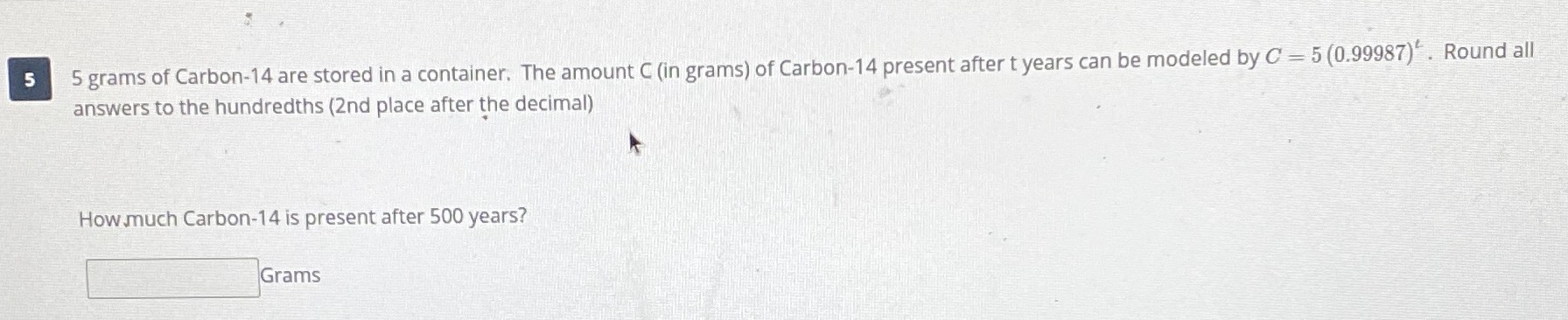### ¿Todavía tienes preguntas de matemáticas?

Pregunte a nuestros tutores expertos
Algebra
Pregunta$$5$$ grams of Carbon- $$14$$ are stored in a container. The amount C (in grams) of Carbon- $$14$$ present after t years can be modeled by $$C = 5 ( 0.99987 ) ^ { t }$$ . Round all answers to the hundredths ( $$2$$ nd place after the decimal)

How much Carbon- $$14$$ is present after $$500$$ years?

$$5 \times ( 0.9987 ) ^ { 500 }$$
$$\log ( 1 + e + \pi ^ { 2 } ) \approx 2.609178677401083$$
$$\sqrt { \frac { 5 e } { 2 } } \approx 2.606857221089719$$